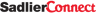Log In

# Catechist Cafe

###### Filter Resources
%} %} %} %} %} %} %} %} %} %} %} %} %} %} %} %} %} %} %} %} %} %} %} %} %}
%} %} %} %} %} %} %} %} %} %} %} %} %} %} %} %} %} %} %}
%} %} %} %} %} %} %} %}
%}
%} %} %} %} %} %} %}
%} %} %} %}
%} %} %} %} %} %}
%}
%}
%} %} %} %} %} %}
%}
%} %} %} %} %} %} %} %} %} %} %} %} %} %} %} %} %} %} %} %} %} %} %} %} %} %} %} %}
%} %} %} %} %} %} %}
%} %} %} %} %} %} %}
%} %} %} %} %} %} %} %} %} %} %} %} %} %} %} %} %} %} %}
%} %} %} %} %} %} %} %} %} %} %} %} %} %} %} %} %} %} %} %} %} %} %} %} %} %} %} %} %} %} %} %} %} %} %} %} %} %} %} %} %} %} %} %} %} %} %} %} %} %} %} %} %} %} %} %} %} %} %} %} %} %} %} %}
%} %} %} %}
%} %} %} %} %} %} %} %} %} %} %} %} %} %} %} %} %} %} %} %} %} %} %} %} %} %} %} %} %} %} %} %} %} %} %} %} %} %} %} %} %} %} %} %} %} %} %} %} %} %} %} %} %} %} %} %} %} %} %} %} %} %} %} %} %} %} %} %} %} %} %} %} %} %} %} %} %} %} %} %} %} %} %} %} %} %} %} %} %} %} %} %} %} %} %} %} %} %} %} %} %} %} %} %} %} %} %} %} %}
%} %} %} %} %} %} %} %} %} %}
%} %} %} %} %} %} %}
%} %} %} %}
%}
%} %} %} %} %} %} %}